# WBJEE 2018 Chemistry Paper with Solutions

WBJEE 2018 Paper with Solutions Chemistry is a perfect resource which helps the candidates in understanding different methods of solving a particular problem. Candidates can download the previous years’ question papers of WBJEE to figure out the frequently occurring questions in the main exams. Practising the WBJEE previous year papers will guide the students to attempt each question with the best possible approach and arrive at the solution as soon as possible to save time. Solving these papers with solutions will help the students understand the concepts and coverage for the upcoming exam.

### WBJEE 2018 - Chemistry

Question 1: Ferric ion forms a Prussian blue precipitate due to the formation of

1. a. K4[Fe (CN)6]
2. b. K3[Fe (CN)6]
3. c. Fe (CNS)3
4. d. Fe4 [Fe (CN)6]3

Solution:

Ferric ion form Prussian blue precipitate due to formation of Fe4[Fe(CN)6]3.

Fe3+ + [Fe(CN)6] –4 → Fe4[Fe(CN)6]3

[Prussian blue]

Question 2: The nucleus 6429Cu accepts an orbital electron to yield.

1. a. 6528Ni
2. b. 6430Zn
3. c. 6428Ni
4. d. 6530Zn

Solution:

29Cu64 + -1eo6428Ni

(k electron capture)

During k- capture mass number remains the same but atomic number decreases by 1 unit for each capture.

Question 3: How many moles of electrons will weigh one kilogram?

1. a. 6.023 × 1023
2. b. (1 / 9.108) × 1031
3. c. (6.023 / 9.108) × 1054
4. d. 1 / [9.108 × 6.023] × 108

Solution:

Mass of one electron = 9.108 × 10–31 kg

So, the mass of one mole of electron = 9.108 × 10–31 × 6.023 × 1023 kg

Then no. of mole of e in 1 kg

= 1 / [9.108 × 6.023 × 10-31 × 1023] = 1 / [9.108 × 6.023] × 108 mole of e

Question 4: Equal weights of ethane and hydrogen are mixed in an empty container at 25°C. The fraction of total pressure exerted by hydrogen is

1. a. 1: 2
2. b. 1 : 1
3. c. 1 : 16
4. d. 15 : 16

Solution:

Given – the weight of ethane = weight of hydrogen gas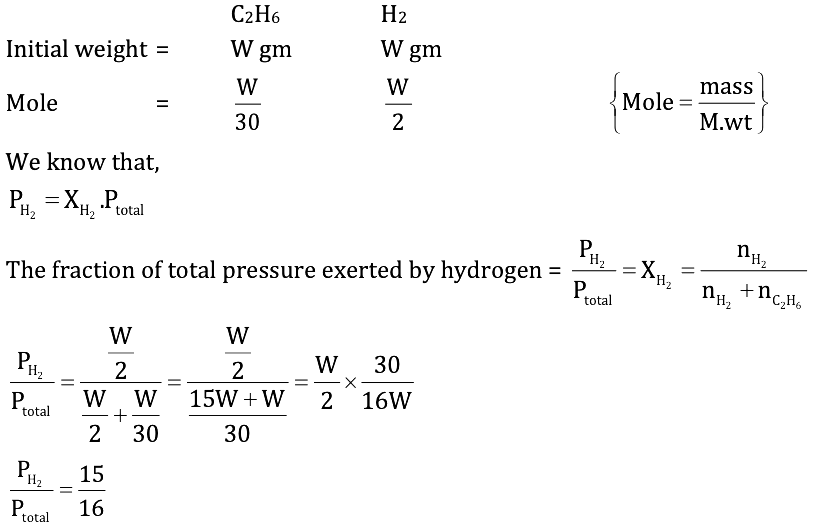Question 5: The heat of neutralization of a strong base and a strong acid is 13.7 kcal. The heat released when 0.6 mole HCl solution is added to 0.25 mole of NaOH is

1. a. 3.425 kcal
2. b. 8.22 kcal
3. c. 11.645 kcal
4. d. 13.7 kcal

Solution:

HCl + NaOH → NaCl + H2O ; ΔH = –13.7 kcal

HCl = 1 mole and NaOH = 1 mole

Now, according to question,

HCl + NaOH → NaCl + H2O

HCl = 0.6 mole and NaOH = 0.25 mole

So, NaOH is limiting reagent,

Then, release energy = 13.7 × 0.25 = 3.425 kcal

Question 6: A compound formed by elements X and Y crystallizes in the cubic structure, where X atoms are at the corners of a cube and Y atoms are at the centres of the body. The formula of the compound is :

1. a. XY
2. b. XY2
3. c. X2Y3
4. d. XY3

Solution:

There are 8 corners in a cube. So, as the X atoms are at corners, so each of the X atoms will contribute 1 / 8 part towards a particular unit cell.

The no. of X atoms per unit cell = 8 * (1 / 8) = 1

Further, the Y atoms are at the centres of the body, so Y atom will contribute 1 part towards a particular unit cell.

The no. of Y atoms per unit cell = 1 × 1 = 1

So, the formula of the compound will be “XY”.

Question 7: What amount of electricity can deposit 1 mole of Al metal at cathode when passed through molten AlCl3?

1. a. 0.3 F
2. b. 1 F
3. c. 3F
4. d. (1 / 3) F

Solution:

AlCl3 → Al3+ + 3Cl

When 1 mole of AlCl3 dissociates, it gives 1 mole of Al3+ and 3 moles of Cl ions. The positively charged aluminium ions move towards a negative electrode or cathode and negatively charged chloride ions will move towards a positively charged electrode or anode.

Reduction (on the cathode):-

Al3+ + 3e → Al

1 mole of aluminium ions will gain 3 moles of electrons to produce 1 mole of aluminium.

1 electron carries charge = 1.6 × 10–19 C

1 mole of electron carries charge = 1.6 × 10–19 × 6.023 × 1023 C = 96500 C and 95600 C = 1 faraday

As, 1 mole of electrons carries 1 faraday.

So, 3 mole of electrons carries 3 faraday of electricity.

Question 8: Given the standard half-cell potentials (E°) of the following as

Zn = Zn2+ + 2e ; E0 = +0.76V

Fe = Fe2+ + 2e ; E0 = 0.41V

Then the standard e.m.f. of the cell with the reaction Fe2+ + Zn → Zn2+ + Fe is

1. a. –0.35V
2. b. + 0.35 V
3. c. +1.17 V
4. d. –1.17 V

Solution: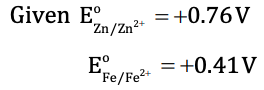The cell reaction –

Fe2+ + Zn → Zn2+ + Fe

At anode (oxidation) – Zn → Zn2+ + 2e ; E° = + 0.76 V

At cathode (reduction) – Fe2+ + 2e → Fe ; E° = –0.41 V

Eocell = SOP of anode + SRP of cathode

= 0.76 + (–0.41) = 0.35 V

Question 9: The following equilibrium constants are given: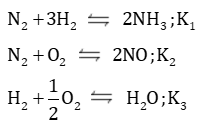The equilibrium constant for the oxidation of 2 mol of NH3 to give NO is

1. a. K1 . (K2 / K3)
2. b. K2 . (K33 / K1) c
3. c. K2 . (K32 / K1)
4. d. K22 . (K3 / K1)

Solution:

According to question,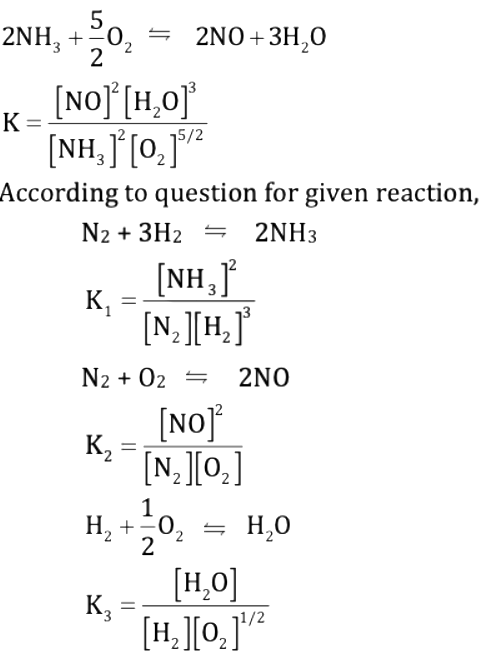On comparing values of K1, K2, K3 with K, we get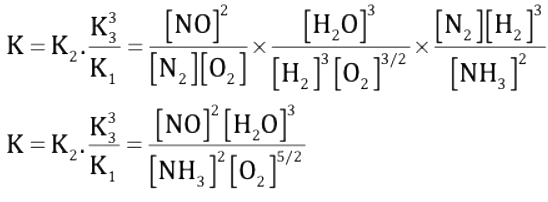Question 10: Which one of the following is a condensation polymer?

1. a. PVC
2. b. Teflon
3. c. Dacron
4. d. Polystyrene

Solution:

Condensation polymers are any kind of polymers formed through a condensation reaction, where molecules join together and losing small molecules as by-products such as water or methanol.

Dacron – It is a condensation polymer of ethylene glycol and terephthalic acid.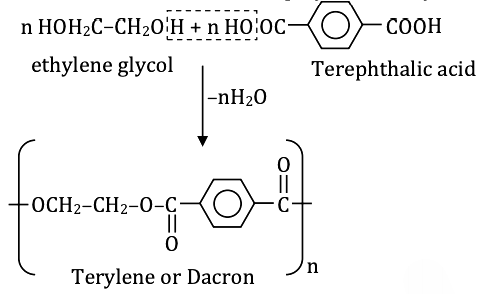Question 11: Which of the following is present in a maximum amount in 'acid rain'?

1. a. HNO3
2. b. H2SO4
3. c. HCl
4. d. H2CO3

Solution:

Sulphuric acid is the main constituent of acid rain because of the following reaction. 2SO2(g) + O2(g) + 2H2O(l) → 2H2SO4(aq.)

Question 12: Which of the set of oxides are arranged in the proper order of basic, amphoteric, acidic?

1. a. SO2, P2O5, CO
2. b. BaO, Al2O3, SO2
3. c. CaO, SiO2, Al2O3
4. d. CO2, Al2O3, CO

Solution:

Basic - BaO

Amphoteric - Al2O3

Acidic - SO2

Question 13: Out of the following outer electronic configurations of atoms, the highest oxidation state is achieved by which one?

1. a. (n - 1) d8 ns2
2. b. (n - 1) d5 ns2
3. c. (n - 1) d3 ns2
4. d. (n - 1) d5 ns1

Solution:

All outer electronic configuration of the element is below to d-block.

So, (n – 1)d5ns2 → [Mn]

Mn show highest oxidation state = +7

Question 14: At room temperature, the reaction between water and fluorine produces

1. a. HF and H2O2
2. b. HF, O2 and F2O2
3. c. F-, O2 and H+
4. d. HOF and HF

Solution: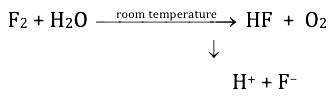Question 15: Which of the following is least thermally stable?

1. a. MgCO3
2. b. CaCO3
3. c. SrCO3
4. d. BeCO3

Solution:

According to Fajan’s rule,

Polarizing power ∝ covalent character ∝ 1 / [size of cation]

And the size of cation ∝ ionic character ∝ thermal stability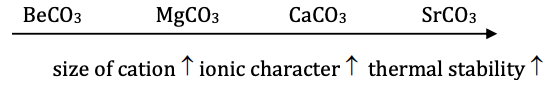So, the least thermal stable is BeCO3.

Question 16: Cl2O7 is the anhydride of

1. a. HOCl
2. b. HClO2
3. c. HClO3
4. d. HClO4

Solution:

Cl2O7 + H2O → 2HClO4 (perchloric acid)

Thus, Cl2O7 is an anhydride of perchloric acid.

Question 17: The main reason that SiCl4 is easily hydrolyzed as compared to CCl4 is that

1. a. Si-Cl bond is weaker than the C-Cl bond.
2. b. SiCl4 can form hydrogen bonds.
3. c. SiCl4 is covalent.
4. d. Si can extend its coordination number beyond four.

Solution:

In SiCl4 vacant d-orbitals are present but in CCl4, C does not have vacant d-orbital. So by hydrolysed SI form co-ordination bond and Si expand its co-ordination number beyond four.

Question 18: Silver chloride dissolves in excess of ammonium hydroxide solution. The cation present in the resulting solution is

1. a. [Ag (NH3)6]+
2. b. [Ag (NH3)4]+
3. c. Ag+
4. d. [Ag (NH3)2]+

Solution:

AgCl + 2NH4OH → [Ag(NH3)2] + Cl + H2O

Silver chloride dissolves in excess of ammonium hydroxide solution to [Ag (NH3)2]+ and Cl.

So the cation is [Ag (NH3)2]+.

Question 19: The ease of hydrolysis in the compounds CH3COCl(I), CH3–CO–O–COCH3(II), CH3COOC2H5(III) and CH3CONH2(IV) is of the order

1. a. I > II > III > IV
2. b. IV > III > II > I
3. c. I > II > IV > III
4. d. II > I > IV > III

Solution:

Reactivity towards hydrolysis of acid derivation is

Acid halide > Anhydride > Ester > Amide

So, CH3COCl > CH3–CO–O–COCH3 > CH3COOC2H5 > CH3CONH2

(I) (II) (III) (IV)

Question 20: CH3 − C ≡ C MgBr can be prepared by the reaction of

1. a. CH3 − C ≡ C – Br with MgBr2
2. b. CH3 − C ≡ CH with MgBr2
3. c. CH3 − C ≡ CH with KBr and Mg metal
4. d. CH3 − C ≡ CH with CH3 MgBr

Solution: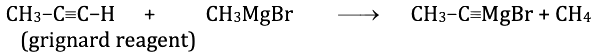Question 21: The number of alkene(s) which can produce-2-butanol by the successful treatment of

(i) B2H6 in tetrahydrofuran solvent and

(ii) alkaline H2O2 solution is

1. a. 1
2. b. 2
3. c. 3
4. d. 4

Solution: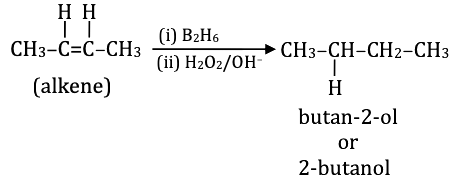Question 22: Identify 'M' in the following sequence of reactions :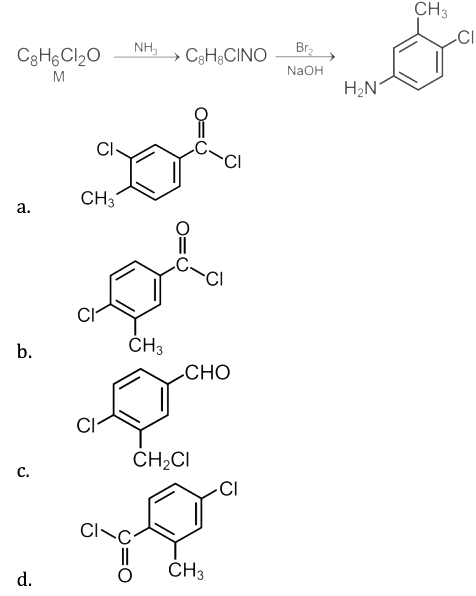Solution: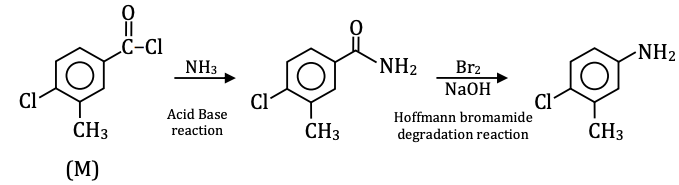Question 23: Methoxybenzene on treatment with HI produces :

1. a. Iodobenzene and methanol
2. b. Phenol and methyl iodide
3. c. Iodobenzene and methyl iodide
4. d. Phenol and methanol

Solution: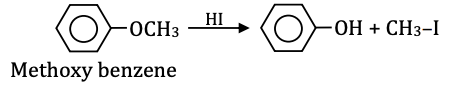Question 24: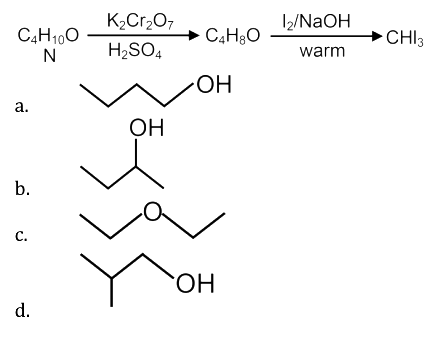Solution: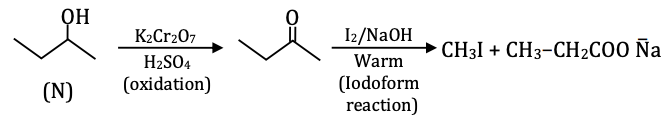Question 25: The correct order of reactivity for the addition reaction of the following carbonyl compounds with Ethyl Magnesium Iodide is :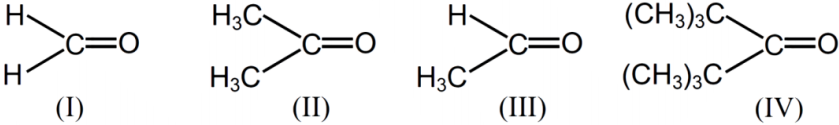1. a. I > III > II > IV
2. b. IV > III > II > I
3. c. I > II > IV > III
4. d. III > II > I > IV

Solution:

Reactivity ∝ 1 / steric crowding

So, the order of nucleophilic addition reaction is,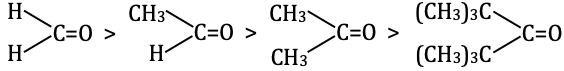Question 26: If aniline is treated with conc. H2SO4 and heated at 200 °C, the product is

1. a. Anilinium sulphate
2. b. Benzenesulphonic acid
3. c. m-Aminobenzenesulphonic acid
4. d. Sulphanilic acid

Solution: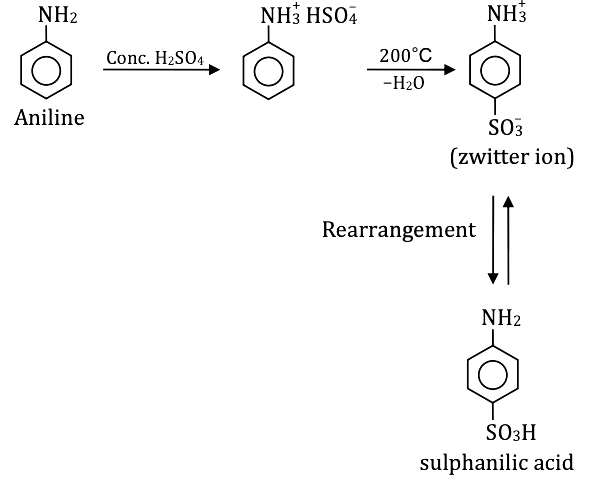Question 27: Which of the following electronic configuration is not possible?

1. a. n = 3, l = 0, m = 0
2. b. n = 3, l = 1, m = – 1
3. c. n = 2, l = 0, m = –1
4. d. n = 2, l = 1, m = 0

Solution:

We know that, if n = a then l = 0 to a – 1 and m = –l to +l

(A) n = 3, l = 0, m = 0, 3S

(B) n = 3, l = 1, m = 1, 3P

(C) n = 2, l = 0, m = –1, (not possible)

(D) n = 2, l = 1, m = 0, 3P

Question 28: The number of unpaired electrons in Ni (atomic number = 28) are

1. a. 0
2. b. 2
3. c. 4
4. d. 8

Solution: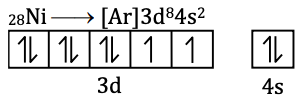No. of unpaired electrons = 2

Question 29: Which of the following has the strongest H-bond?

1. a. O – H … S
2. b. S – H … O
3. c. F – H … F
4. d. F – H … O

Solution:

Due to the highest electronegativity of F, it is more polar, so it is formed stronger H– bonding.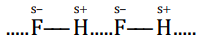Question 30: The half-life of C14 is 5760 years. For a "200" mg sample of C14, the time taken to change to 25 mg is

1. a. 11520 years
2. b. 23040 years
3. c. 5760 years
4. d. 17280 years

Solution: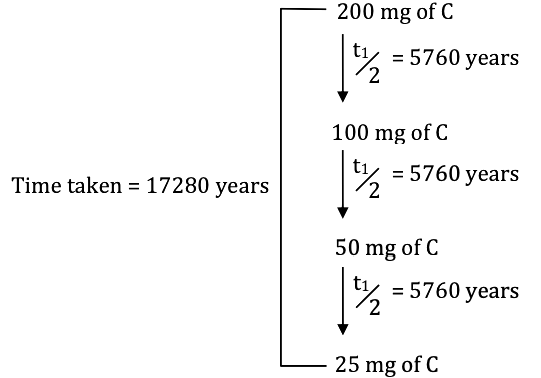It takes 3 half–life to reduce 200 mg of C14 to 25 mg i.e. = 3 × 5760 = 17280 years.

Question 31: During a reversible adiabatic process, the pressure of a gas is found to be proportional to the cube of its absolute temperature. The ratio CP / Cv for the gas is

1. a. 3 / 2
2. b. 7 / 2
3. c. 5 / 3
4. d. 9 / 7

Solution:

PTγ/1-γ = constant, for the reversible adiabatic process.

Given –

P ∝ T3

PT-3 = constant

On comparing,

γ / [1 - γ] = -3

γ = –3 + 3γ

2γ = 3

γ = 3 / 2

γ = CP / Cv

CP / Cv = 3 / 2

Question 32: [X] dil.H2SO4 → [Y] : Colourless, suffocating gas [Y] K2Cr2O7 + H2SO4 → Green colouration of solution Then, [X] and [Y] are

1. a. SO32-, SO2
2. b. Cl, HCl
3. c. S2-, H2S
4. d. CO32-, CO2

Solution: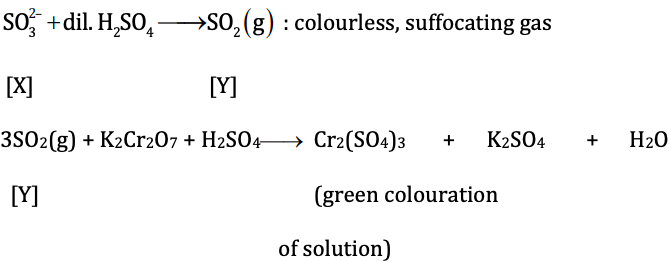Question 33: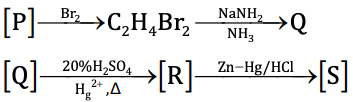The species P, Q, R and S respectively are

1. a. ethene, ethyne, ethanal, ethane
2. b. ethane, ethyne, ethanal, ethene
3. c. ethene, ethyne, ethanal, ethanol
4. d. ethyne, ethane, ethene, ethanal

Solution: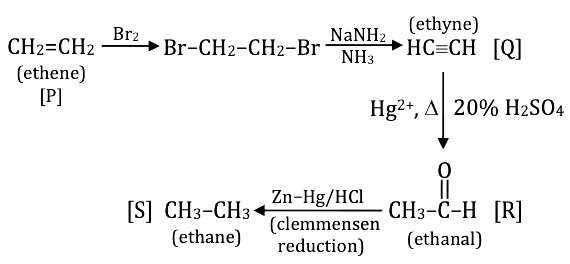Question 34: The number of possible organobromine compounds which can be obtained in the allylic bromination of 1–butene with N–bromosuccinimide is

1. a. 1
2. b. 2
3. c. 3
4. d. 4

Solution: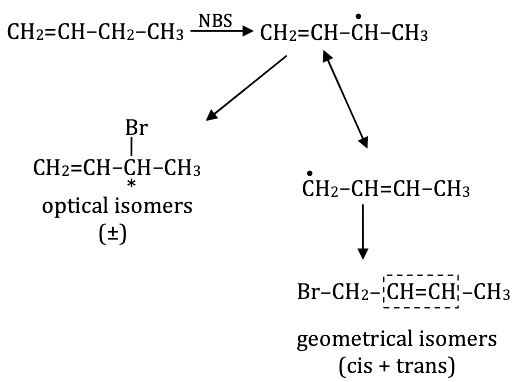So, the total number of possible organobromine compounds = 4.

Question 35: A metal M (specific heat 0.16) forms a metal chloride with ≈ 65% chlorine present in it. The formula of the metal chloride will be

1. a. MCl
2. b. MCl2
3. c. MCl3
4. d. MCl4

Solution:

Let, the formula of metal chloride is MClx then,

We know that,

Atomic weight of metal = 6.4 / specific heat

Atomic weight of metal = [6.4 / 0.16] = 40

According to question –

% of Cl = 65% in metal chloride

% of Cl = [mass of Cl in compound / Total mass of compound] * 100

65 = {[35.5 * x] / [40 + 35.5x]} * 100

x = 2.09 ≈ 2

x = 2 approx.

So, metal chloride MClx = MCl2

Question 36: Among the following, the extensive variables are

1. a. H (Enthalpy)
2. b. P (Pressure)
3. c. E (Internal energy)
4. d. V (Volume)

Solution:

Extensive variable → H(enthalpy), E(Internal energy) and V(volume),

Since variable, depends on the amount of substance or volume or size of the system.

Question 37: White phosphorus P4 has the following characteristics :

1. a. 6 P – P single bonds
2. b. 4 P – P single bonds
3. c. 4 lone pair of electrons
4. d. P – P – P angle of 60°

Solution:

1. Answer: (a, c, d)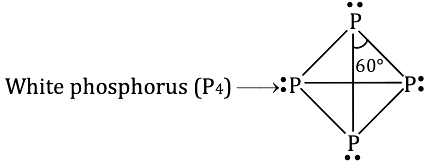(A) 6 P–P single bond

(B) 4 lone pair of electrons

(D) Bond angle < P–P–P = 60°

Question 38: The possible product (s) to be obtained from the reaction of cyclobutyl amine with HNO2 is/are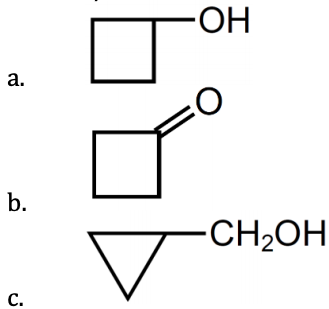1. d. H2C = CH2

Solution:

1. Answer: (a, c)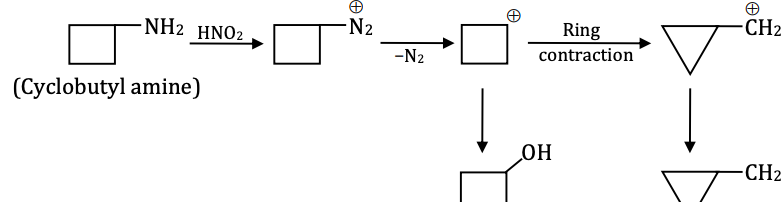Question 39: The major product(s) obtained in the following reaction is/are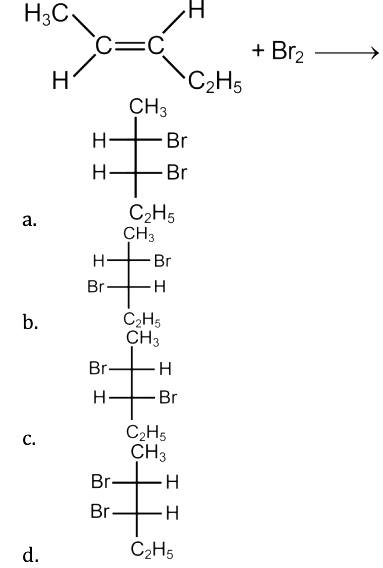Solution:

1. Answer: (a, d)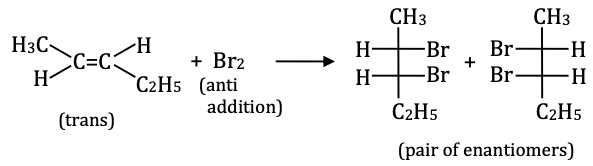Question 40: Which statements are correct for the peroxide ion?

1. a. It has five completely filled anti-bonding molecular orbitals.
2. b. It is diamagnetic
3. c. It has a bond order one.
4. d. It is isoelectronic with neon

Solution:

1. Answer: (b, c)

O2-2 → (Peroxide ion) = 18e

By MOT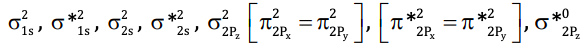B.O. = [Nb - Na] / 2 , Nb = No. of bonding eand Na = No. of antibonding e

B.O. = [10 – 8] / 2 = 1

No. of unpaired electron = 0 (diamagnetic)

### WBJEE 2018 Chemistry Question Paper with Solutions